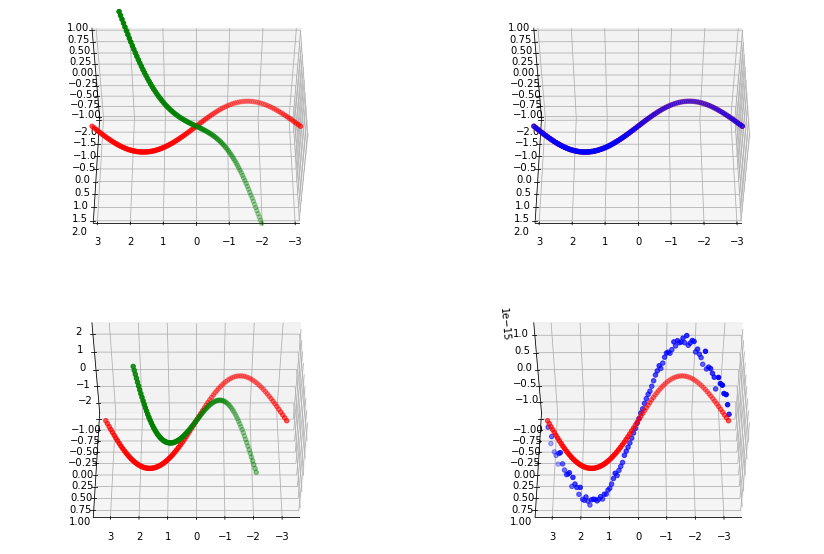# The Kabsch AlgorithmTop left we show the original points in red and the rotated points in green. On the top right we see the original points in red and the rectified points in blue. Note that due to the similarity, this plot appears to only show one line. On the images in the bottom row, we show the same information as in the first row, but allow the plotting library to determine the appropriate plotting scale.

In this post, I finally cover the Kabsch algorithm which can be used to retrieve the rigid body transform between two sets of points (with known 1-to-1 correspondences).

The algorithm is pretty straightforward and I've actually just followed the wikipedia description almost word for word: https://en.wikipedia.org/wiki/Kabsch_algorithm

In the toy example, we generate two matrices P and Q where each row of the matrix corresponds the coordinates of a 3-D point. Note that in this algorithm we assume that the correspondences between the two sets of points are perfectly known. In this case, each row in Q represents the rotated version of the point on the same row in P.

In an upcoming post I will be looking at alternative to the Kabsch algorithm. For now, here's my quick implementation of the Kabsch algorithm, tested using the random rotation matrix generation method I covered previously in https://fulkast.medium.com/from-graham-schmidt-to-so-n-ca7ab00d1624

Apart from the visual verification of the calculated rotation matrix, notice that the difference between the original rotation matrix and the estimated rotation matrix is very close to identity (the zero element in SO(3)).

I hope this quick implementation is helpful ;)